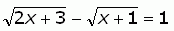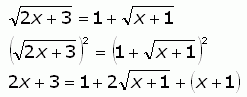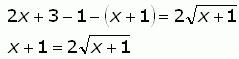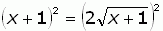SEARCH HOMEMath Central Quandaries & QueriesQuestion from JC, a student: I have a difficult time trying to work this problem. This is algebra math. The problem is about Sloving Equations with Radicals, using the power rule ; squaring twice. radical 2x+3- radical x+1= 1 the answer is 3, -1.Hi JC.

Let me first re-write your equation:The first step is to isolate one of the radical terms. I'll choose to isolate the first radical term. Then you can square both sides.Do you see how this has eliminated one of the radicals? Now I re-arrange to isolate the other radical term.I don't need to move the 2 over (but I could) because it is just a factor of the radical. Now I square both sides again:When I do this, and simplify, I get a quadratic equation that I can easily factor (if not, I could use the quadratic formula).

Hope this helps,
Stephen La Rocque.Math Central is supported by the University of Regina and The Pacific Institute for the Mathematical Sciences.